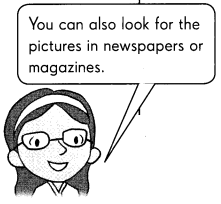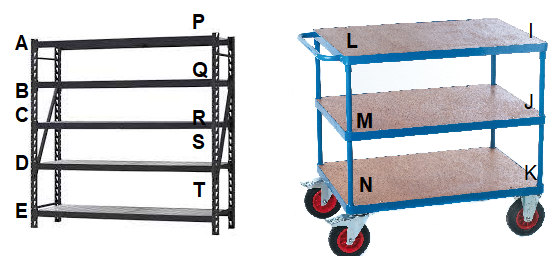# Math in Focus Grade 3 Chapter 17 Practice 4 Answer Key Parallel Lines

This handy Math in Focus Grade 3 Workbook Answer Key Chapter 17 Practice 4 Parallel Lines provides detailed solutions for the textbook questions.

## Math in Focus Grade 3 Chapter 17 Practice 4 Answer Key Parallel Lines

Guess if the lines are parallel. Count square units between the lines to check your guess.

Question 1.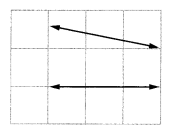Lines are not parallel
Explanation: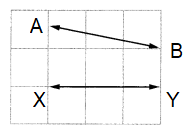The distance between AX is 1.5 square units and BY is 1 square unit,
distance between the lines AB and XY,
if we extend the lines, they meet at one end, So, the given lines are not parallel lines.

Question 2.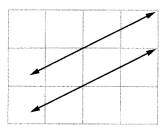lines are parallel lines
Explanation: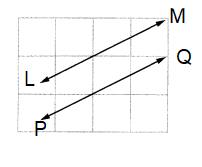LM and PQ are parallel lines and the distance between the lines is more then half square meter,
the lines LM and PQ never meet at any point if we extend.

Question 3.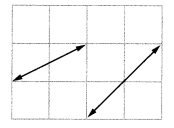lines are not parallel lines.
Explanation: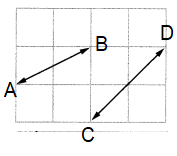The distance between AB is 105 square units and BY is 1 square unit distance between the lines AB and XY
if we extend the lines, they meet at one end.
So, the given lines are not parallel lines.

Question 4.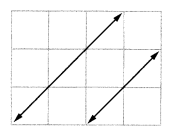Lines are parallel lines.
Explanation: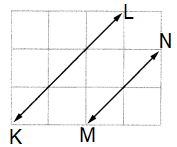KL and MN are parallel lines and the distance between the lines is one square meter,
the lines KL and MN never meet at any point if we extend.
So, the given lines are parallel.

Question 5.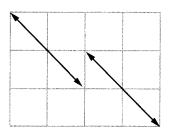Lines are parallel lines.
Explanation: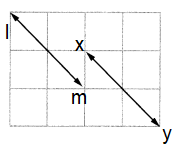lm and xy are parallel lines and the distance between the lines is half square meter,
the lines lm and xy never meet at any point if we extend.
So, the given lines are parallel.

Question 6.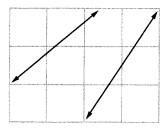Lines are not parallel lines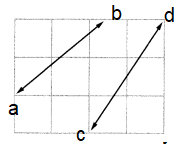Explanation:
If we extend the lines, they meet at one end,
So, the given lines are not parallel lines.

Check (✓) the box if the lines are parallel.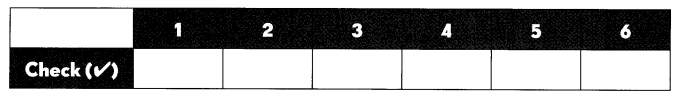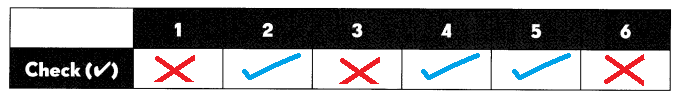Explanation:
From the above figures we can come to conclusion which figures are parallel lines and which are not parallel lines.

Count square units between lines to find parallel lines. Use a colored pencil to trace a pair of parallel fines in each figure.

Question 7.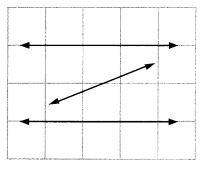2 square units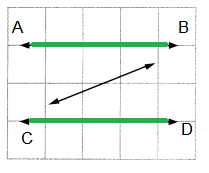Explanation:
AB and CD are parallel lines and
The distance between AB and CD are 2 square units.

Question 8.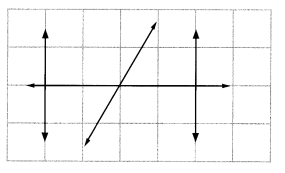4 square units between the parallel lines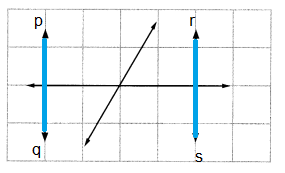Explanation:
pq and rs are parallel lines and
The distance between pq and rs are 4 square units.

Question 9.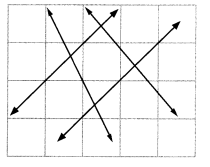one square unit between the wx & yz parallel lines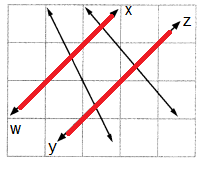Explanation:
wx and yz are parallel lines and
The distance between wx and yz are 1 square units.

Question 10.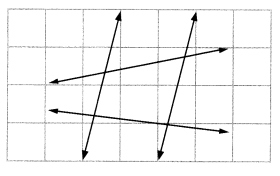2 square units between the parallel lines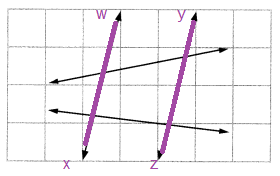Explanation:
wx and yz are parallel lines and
The distance between wx and yz are 2 square units.

Circle the letters that have parallel line segments.

Question 11.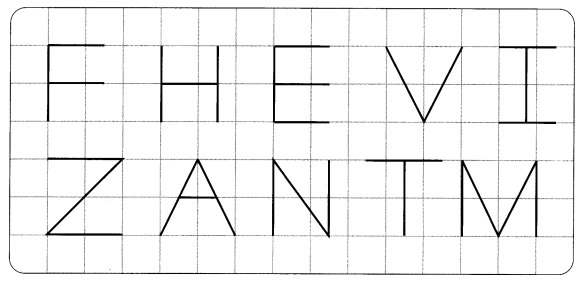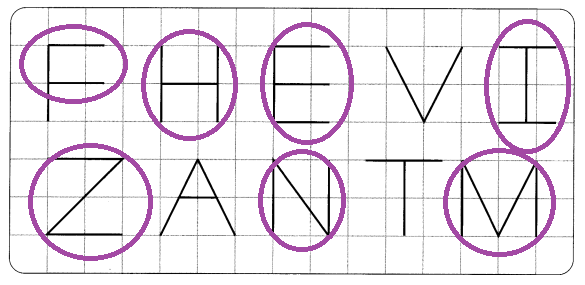Explanation:
Two lines are said to be parallel when they do not meet at any point in a plane.
Letters F, H, E, I, Z, N and M have parallel lines and are circled.
Letters V, A and T hand no parallel lines.

Name all the pairs of parallel line segments in each figure.

Example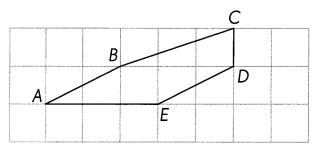Segments AB and ED

Question 12.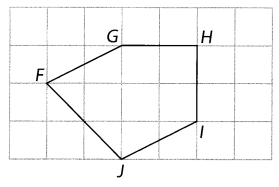Segments FG and JI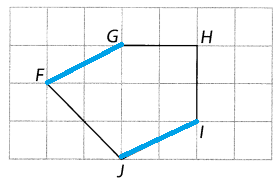Explanation:
Two lines are said to be parallel when they do not meet at any point in a plane.
So, Segments FG and JI are paraell.

Question 13.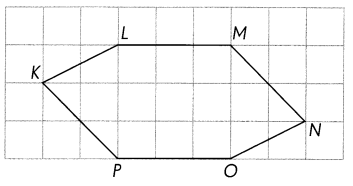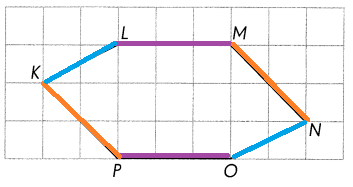Segments PK and NM
Segments LM and PO
Segments KL and ON
Explanation:
Parallel Lines or parallel Segments are always the same distance apart, they will never meet.
So, the above all are parallel.

Question 14.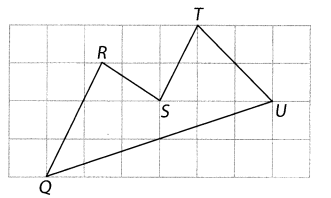Segments RQ and TS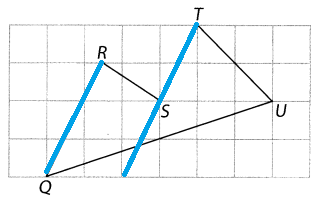Explanation:
Two lines are said to be parallel when they do not meet at any point in a plane.
So, Segments RQ and TS are paraell.

Identify and name a pair of parallel line segments on each object.

Question 15.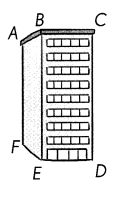Segments AB and FE
Segments BC and ED
Segments AF and BE
Segments CD and BE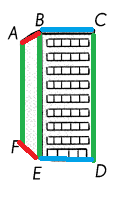Explanation:
Parallel Lines or parallel Segments are always the same distance apart, they will never meet.
So, the above all are parallel.

Question 16.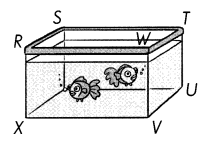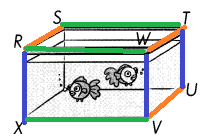Segments RX and WV
Segments WV and TU
Segments WT and VU
Segments RS and WT
Segments WT and VU
Explanation:
Parallel Lines or parallel Segments are always the same distance apart, they will never meet.
So, the above all are parallel.

Carry out this activity.

Question 17.
Think of two objects that have parallel line segments. Use the Internet to find pictures of these objects. Print and paste them below. Use a colored pencil to trace a pair of parallel line segments on each object.